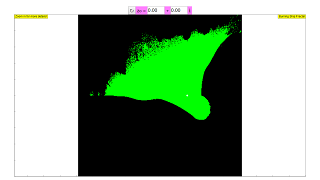Introduction

# The Burning Ship Fractal

The Burning Ship fractal consists of points, c, in the complex plane that obey the following rule

1. Start with the complex number z = a + ib where a and b are real numbers.
2. Generate a new complex number, z', by taking the absolute value of both the real and imaginary parts before squaring the resulting complex number, and adding the result to c. z' = (|Re(z)| + |Im(z)|*i)2 + c.
3. Repeat steps 1 and 2. If the complex number z goes toward infinity, then the starting point, c, is not a member of the Mandelbrot set. All numbers that remain bounded are members of the set.
It can be shown that if the magnitude of z is greater than 2 , then z will approach infinity. The code assumes that the number c is in the set if |z| > 2 after 256 iterations. In order to show how rapidly a number fails the test, we color the pixel corresponding to the number of iterations. Extension

# Mandelbrot set with variable initial value

The members of the Mandelbrot set follow the rule

zn+1= zn2+ c ; initial value z0 = 0

c is a complex number, as is z

We look for points c in the complex plane, for which the series does not diverge. They form the Mandelbrot set. In the simulation they are colored red, lying within a range with the fractal boundary, the famous "apple man". Diverging points are colored green to blue, with the color shading indicating the speed of divergence.

Drawing a rectangle with the mouse defines a smaller range of calculation with correspondingly increasing resolution. Reset leads back to the initial condition.

The series has the members 0, c, c2+ c, c4 + 2c3 + c2 + c,...

For deeper understanding this simulation modifies the series in such a way, that the initial zo value is variable:

z0 = real(z0) + i * im(z0) = k + i m

This series has the members z0 , z02+c, ( z02+c)2+c, , ...

z0 = 0 (k = 0 , m = 0) delivers the common Mandelbrot set, which is the default case for the simulation.

In the drawing z0 corresponds to the white point, which can be drawn with the mouse. The fractal changes according to the change of the initial value. The values of the real and the imaginary part of z0 are shown in two editable number fields. There one can input exact values (press the ENTER key after every change).

The fractal structure is caused by the nonlinearity of the series rule. It is observed for other nonlinear series as well, with different structure of the fractal.

Authors mandelbrot.xlm was originally developed by Francisco Esquembre and Wolfgang Christian The variations were developed by Dieter Roess in October 2008

This simulation is part of

– Plus 2000 Examples from Physics”

ISBN 978-3-11-025005-3, Walter de Gruyter GmbH & Co. KG

### Translations

Code Language Translator Run### Software Requirements

SoftwareRequirements

 Android iOS Windows MacOS with best with Chrome Chrome Chrome Chrome support full-screen? Yes. Chrome/Opera No. Firefox/ Samsung Internet Not yet Yes Yes cannot work on some mobile browser that don't understand JavaScript such as..... cannot work on Internet Explorer 9 and below

### CreditsDieter Roess - WEH- Foundation; Tan Wei Chiong; Loo Kang Wee; Francisco Esquembre; Wolfgang Christian

[text]

### For Teachers

The Burning Ship fractal is actually a slight modification to the Mandelbrot Set by taking the absolute value of both the real and imaginary parts of the complex number z before squaring it in each iteration.

Thus, the equation is as follows:While this may seem like a small change to the equation, the change to the fractal itself is anything but.

Research

[text]

[text]

### Version:

1. http://weelookang.blogspot.sg/2016/02/vector-addition-b-c-model-with.html improved version with joseph chua's inputs
2. http://weelookang.blogspot.sg/2014/10/vector-addition-model.html original simulation by lookang

[text]